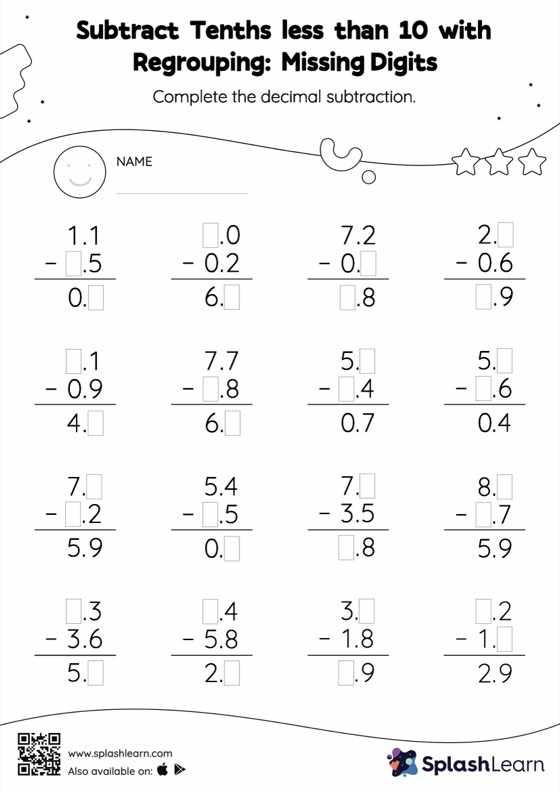# Subtract Tenths less than 10 with Regrouping: Missing Digits Worksheet

Home > Subtract Tenths less than 10 with Regrouping: Missing DigitsThis worksheet gives students an opportunity to apply the concepts of subtraction to subtract tenths less than 10 with regrouping. Students use the relationship between addition and subtraction to find the missing number while subtracting decimals. To reach the answer, they must also regroup the numbers in subtract tenths less than 10 with regrouping worksheet using the relationships between ones, tenths, hundredths, thousandths, etc.